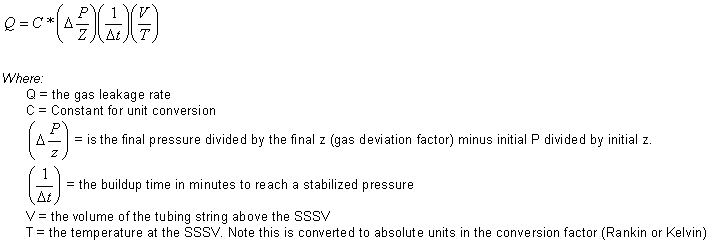Well Integrity Tracking System Gas Leak Rate Calculator Notes   User Name:
Company:
Well:

Gas Leak Rate Calculator Notes
The code for this program is based on equations provided in API 14B, "Design, Installation, Repair and Operation of Subsurface Safety Valve Systems", section E.1.7, Fifth edition, October 2005.

Caution: API RP 14B 5th edition contains an error - the conversion factor for SI units is low by a factor of 100 and underestimates the actual leak rate. The correct units for the particular conversion factors are given in the table below.

Limitations to this approach include:
• Surface valves can leak
• Wellbore cooling from shutting in the well
• Jewel-Thompson effect across SSSV introduces cooling
• Wet gas composition
• Fluid in production stream separates out, altering volume
• Pressure below SSSV continues to build during testInputs

 SI English Notes Q - leakage rate m3/min scf/min Allowable leak rate (API 14B, appendix E) SI units: .43 m3/min, or 400 cm3/min liquidEnglish units: 15 scf/min, or 13.5 oz/min liquid C - unit conversion factor 2840 35.37 Note the coefficient for SI units is different from API RP14B. 14B is low by a factor of 100 based on the specified units. P - pressure MPa psi Range is 0 psig to 10,000 psig. Note: the final pressure must be great than or equal to the initial pressure.Note: 1 MPa = 10 bar = 1,000,000 Pa z - gas deviation factor The technique used by this program was developed by Dranchuk, Purvis and Robinson. The Benedict-Webb-Rubin equation of state was fit to the Z factor surface defined by the Standing-Katz Z factor correlation. The resulting non-linear equation is solved for Z as a function of reduced temperature and pressure using Newton-Raphson iteration. t - build-up time minutes minutes V - volume, amount of gas space m3 ft3 In deepwater applications, the SSSV may be closed, then the tubing above the SSV partially loaded with Methanol to reduce the volume to be pressured and reduce the test length. The volume in this case is the remaining gas volume after the methanol has been pumped into the well. T - temperature C F Range is -20F to 300F Note: the inputs for the program are in C or F. The program converts to absolute values. Note: the following are used by the program to calculate the z factors. Gas Gravity Default value of gas gravity is .6 (air = 1.0). Gas gravity must be between 0.55 and 1.10. CO2 Range is 0-80%. The sum of CO2, H2S and N2 must be less than 100%. The sum of CO2 and H2S must be less than 80% H2S Range is 0-80%. N2 Range is 0-100%.

Contact Information